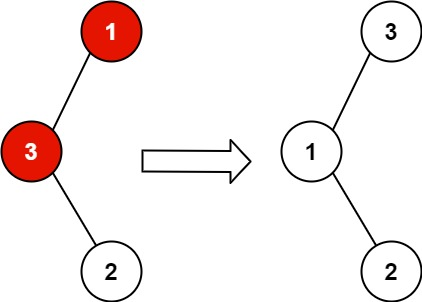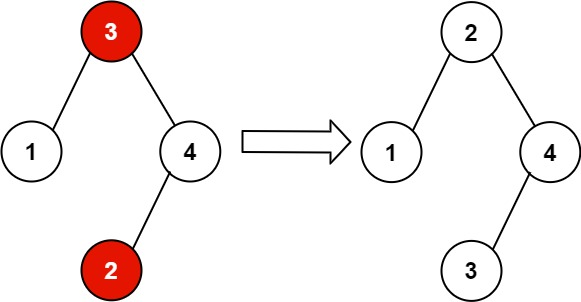304 North Cardinal St.
Dorchester Center, MA 02124

# Recover Binary Search Tree LeetCode Solution

## Problem – Recover Binary Search Tree LeetCode Solution

You are given the `root` of a binary search tree (BST), where the values of exactly two nodes of the tree were swapped by mistake. Recover the tree without changing its structure.

Example 1:``````Input: root = [1,3,null,null,2]
Output: [3,1,null,null,2]
Explanation: 3 cannot be a left child of 1 because 3 > 1. Swapping 1 and 3 makes the BST valid.
``````

Example 2:``````Input: root = [3,1,4,null,null,2]
Output: [2,1,4,null,null,3]
Explanation: 2 cannot be in the right subtree of 3 because 2 < 3. Swapping 2 and 3 makes the BST valid.
``````

Constraints:

• The number of nodes in the tree is in the range `[2, 1000]`.
• `-231 <= Node.val <= 231 - 1`

Follow up: A solution using `O(n)` space is pretty straight-forward. Could you devise a constant `O(1)` space solution?

## Recover Binary Search Tree LeetCode Solution in Python

``````class TreeNode:
def __init__(self, val, left=None, right=None):
self.val = val
self.left = left
self.right = right
def __repr__(self):
return 'TreeNode({})'.format(self.val)

def deserialize(string):
if string == '{}':
return None
nodes = [None if val == 'null' else TreeNode(int(val))
for val in string.strip('[]{}').split(',')]
kids = nodes[::-1]
root = kids.pop()
for node in nodes:
if node:
if kids: node.left  = kids.pop()
if kids: node.right = kids.pop()
return root

def drawtree(root):
def height(root):
return 1 + max(height(root.left), height(root.right)) if root else -1
def jumpto(x, y):
t.penup()
t.goto(x, y)
t.pendown()
def draw(node, x, y, dx):
if node:
t.goto(x, y)
jumpto(x, y-20)
t.write(node.val, align='center', font=('Arial', 12, 'normal'))
draw(node.left, x-dx, y-60, dx/2)
jumpto(x, y-20)
draw(node.right, x+dx, y-60, dx/2)
import turtle
t = turtle.Turtle()
t.speed(0); turtle.delay(0)
h = height(root)
jumpto(0, 30*h)
draw(root, 0, 30*h, 40*h)
t.hideturtle()
turtle.mainloop()

if __name__ == '__main__':
drawtree(deserialize('[1,2,3,null,null,4,null,null,5]'))
drawtree(deserialize('[2,1,3,0,7,9,1,2,null,1,0,null,null,8,8,null,null,null,null,7]'))
``````

## Recover Binary Search Tree LeetCode Solution in C++

``````class Solution {
public:
TreeNode* firstMistake, *secondMistake, *pre;
void recoverTree(TreeNode* root) {
pre = new TreeNode(INT_MIN);
inorder(root);
swap(firstMistake->val, secondMistake->val);
}

void inorder(TreeNode* root) {
if(root == nullptr)
return;

inorder(root->left);

if(firstMistake == nullptr && root->val < pre->val)
firstMistake = pre;
if(firstMistake != nullptr && root->val < pre->val)
secondMistake = root;
pre = root;

inorder(root->right);
}
};
``````

## Recover Binary Search Tree LeetCode Solution in Java

``````class Solution {
TreeNode prev = null, first = null, second = null;

public void recoverTree(TreeNode root) {
evalSwappedNodes(root);
int temp = first.val;
first.val = second.val;
second.val = temp;
}

private void evalSwappedNodes(TreeNode curr) {
if (curr == null)
return;
evalSwappedNodes(curr.left);
if (prev != null && prev.val > curr.val) {
if (first == null)
first = prev;
second = curr;
}
prev = curr;
evalSwappedNodes(curr.right);
}
}
``````
##### Recover Binary Search Tree LeetCode Solution Review:

In our experience, we suggest you solve this Recover Binary Search Tree LeetCode Solution and gain some new skills from Professionals completely free and we assure you will be worth it.

If you are stuck anywhere between any coding problem, just visit Queslers to get the Recover Binary Search Tree LeetCode Solution

Find on Leetcode

##### Conclusion:

I hope this Recover Binary Search Tree LeetCode Solution would be useful for you to learn something new from this problem. If it helped you then don’t forget to bookmark our site for more Coding Solutions.

This Problem is intended for audiences of all experiences who are interested in learning about Data Science in a business context; there are no prerequisites.

Keep Learning!

More Coding Solutions >>

LeetCode Solutions

Hacker Rank Solutions

CodeChef Solutions